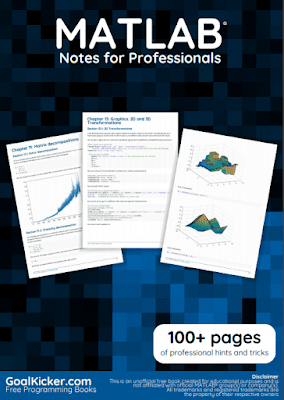This book has 100+ pages that cover the basic to advanced topic of MATLAB programming with practical program and code examples and developed by www.goalkicker.com. if you want to learn MATLAB programming then this book is for you. this book is developed by professional programmers for educational purposes.

### Book contents

1. Getting started with MATLAB language
2. initializing matrices or arrays
3. conditions
4. functions
5. set operations
6. documenting functions
7. using functions with logical output
8. for loops
9. object-oriented programming
10. vectorization
11. matrix decompositions
12. Graphics 2D and 3D transformations
13. controlling subplot coloring in MATLAB
14. image processing
15. drawing
16. financial application
17. Fourier transforms and inverse Fourier transforms
18. ordinary differential equations solvers
19. interpolation with MATLAB
20. integration